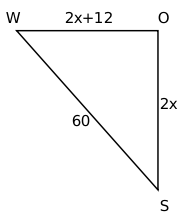Question 52

# Two ships meet mid-ocean, and then, one ship goes south and the other ship goes west, both travelling at constant speeds. Two hours later, they are 60 km apart. If the speed of one of the ships is 6 km per hour more than the other one, then the speed, in km per hour, of the slower ship is

Solution

Let the speeds of two ships be 'x' and 'x+6' km per hour

Distance covered in 2 hours will be 2x and 2x+12It is given,

$$\left(2x\right)^2+\left(2x+12\right)^2=60^2$$

$$\left(x\right)^2+\left(x+6\right)^2=30^2$$

$$2x^2+12x+36=900$$

$$x^2+6x+18=450$$

$$x^2+6x-432=0$$

Solving, we get x = 18

The speed of slower ship is 18 kmph

### View Video Solution

• All Quant CAT Formulas and shortcuts PDF
• 30+ CAT previous papers with solutions PDF